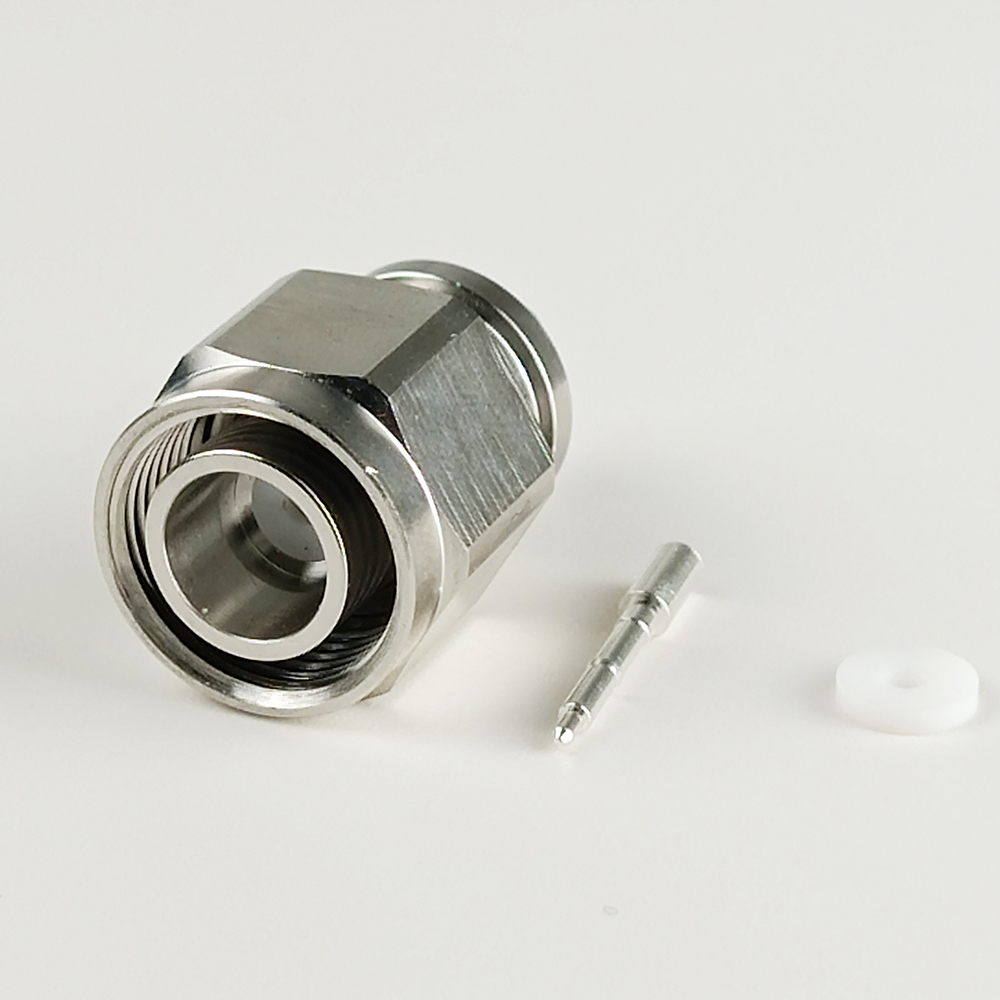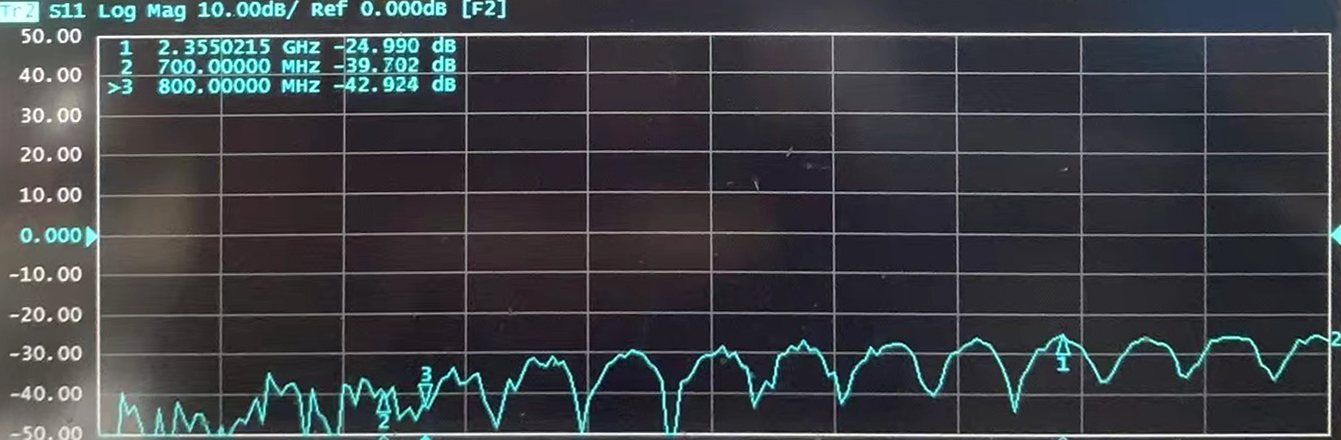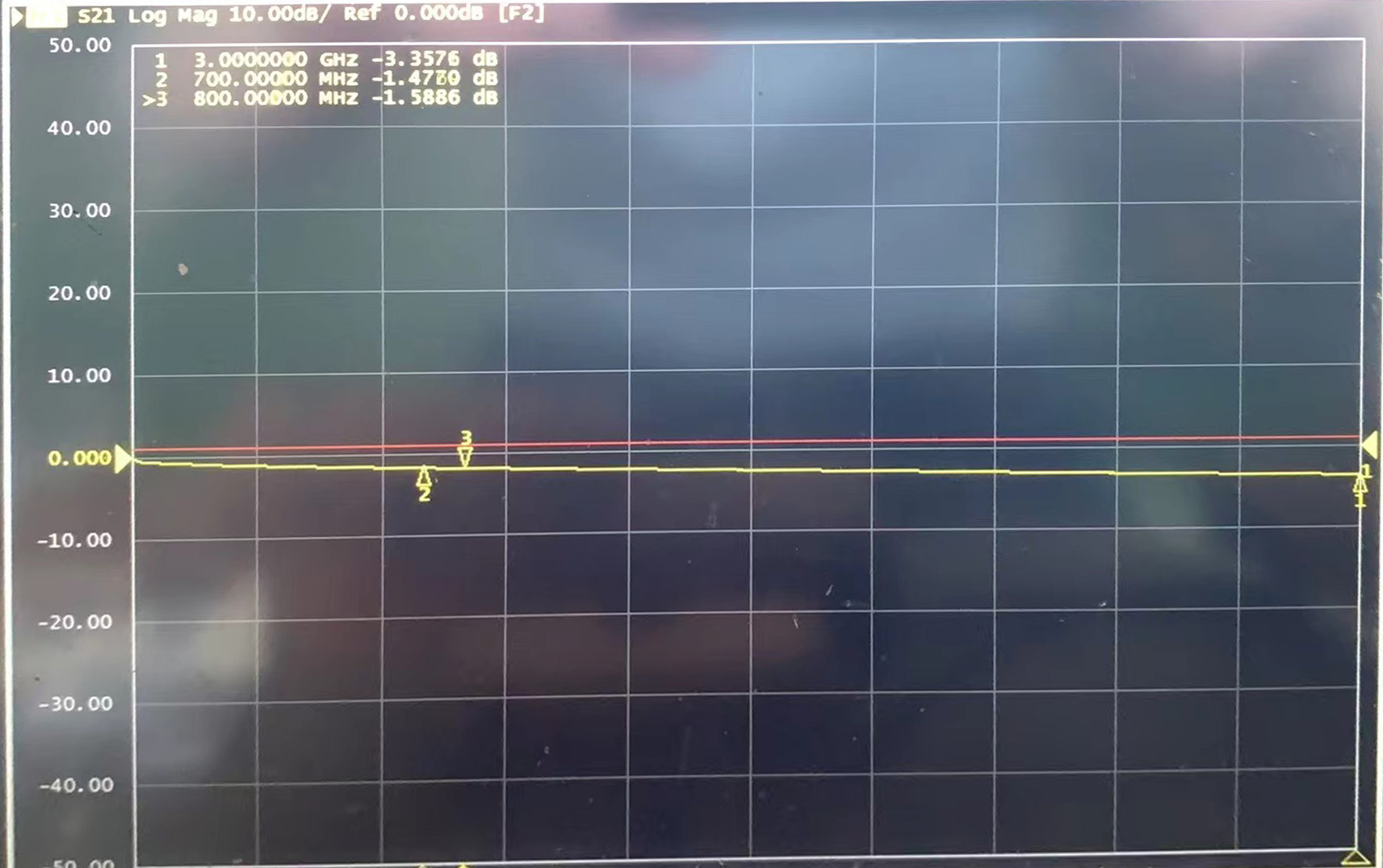Raynool Blog
By Tony Huang | 22 February 2023 | 0 Comments

# Return Loss And Insertion Loss About The RF Connector

Return loss, VSWR and Insertion loss about the RF connector

Return loss and VSWR
Return Loss is one of the characteristic which is easily neglect when discussing about the electric performance of the connector, return loss feature the consistence of cable & connector, it can also express with the VSWR(Voltage Standing Wave Ratio), the ratio of the input to the reflected signal.

The return loss of a system is defined as the ratio of incident power to reflected power and is measured in dB. High return loss results in low reflected power, so high return loss is desirable for a system.

VSWR (Voltage Standing Wave Ratio) measures the efficiency of power transferred from a source to a load. Impedance mismatch is the main cause for the VSWR.

For an ideal system, there is no impedance mismatch, Thus, for this system, VSWR = 1:1, VSWR = 1.00, reflected power is zero, so return loss is infinity

Insertion Loss
Insertion loss means the loss of energy.
While the connector attach to the cable, insertion loss is considered, normally, the insertion loss which is contributed by the connector is very small.
When discussing cable individually, the insertion loss refers to the cable attenuation.

What is the negative(minus) means

There is always a negative (minus) before the figure of return Loss & Insertion Loss, is the meaning the same?

Both return Loss & Insertion Loss have the negative, but the meaning of negative is different, the return loss negative is the result of caculation, the insertion loss negative means the loss of energy.Return LossInsertion Loss